# Moo Tracker

A Wi-Fi Cow Collar that measures the daily movements of a cow and determine its usual temperature, movements and feeding time.

IntermediateFull instructions provided4 days3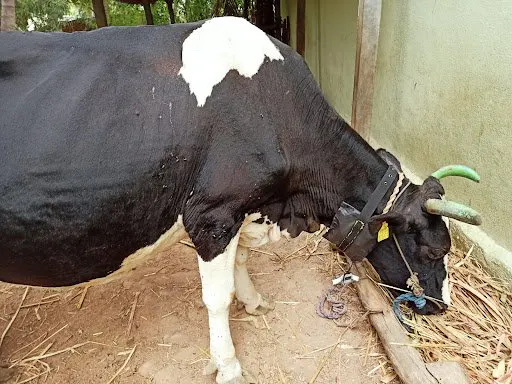## Things used in this project

### Hardware componentsParticle Photon
×1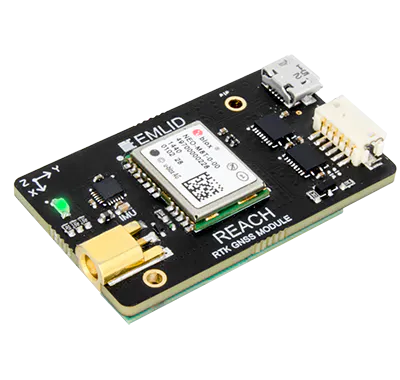×1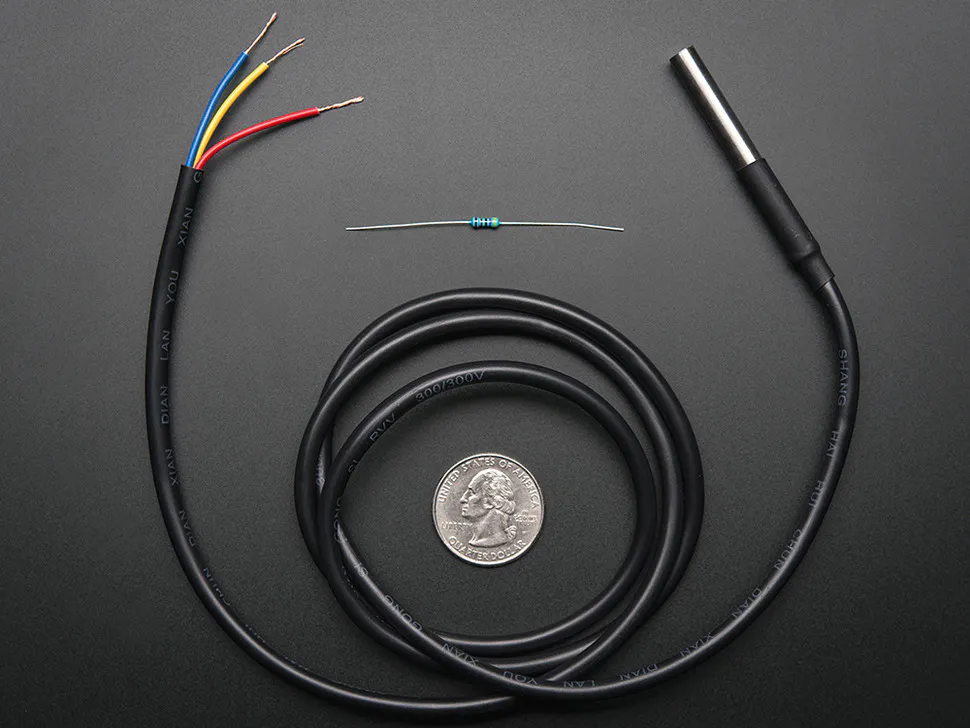Adafruit Waterproof DS18B20 Digital temperature sensor
×1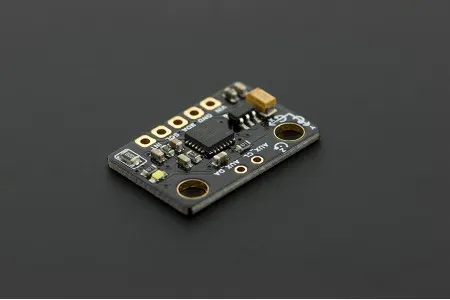DFRobot 6 DOF Sensor - MPU6050
×1
 MAX9814 Module
×1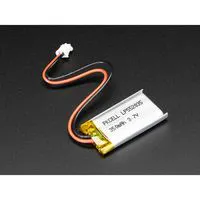Battery, 3.7 V
×1

### Software apps and online servicesParticle Build Web IDEUbidots

### Hand tools and fabrication machines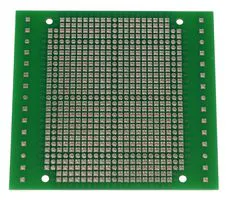PCB, For DMB-4775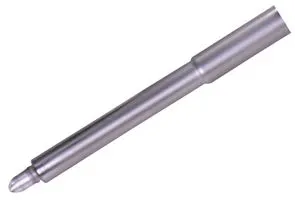Power Head Tip, for use with GT7A Soldering GunSoldering iron (generic)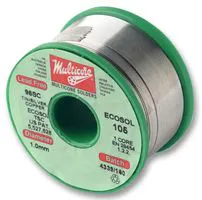Wire Stripper & Cutter, 22-10 AWG / 0.64-2.6mm Capacity Single & Stranded WiresDrill / Driver, 20V
 angle grinder

## Schematics

### Schematics of the Prototype

Basic Interfacing o several Sensors onto the Particle Photon Dev-Board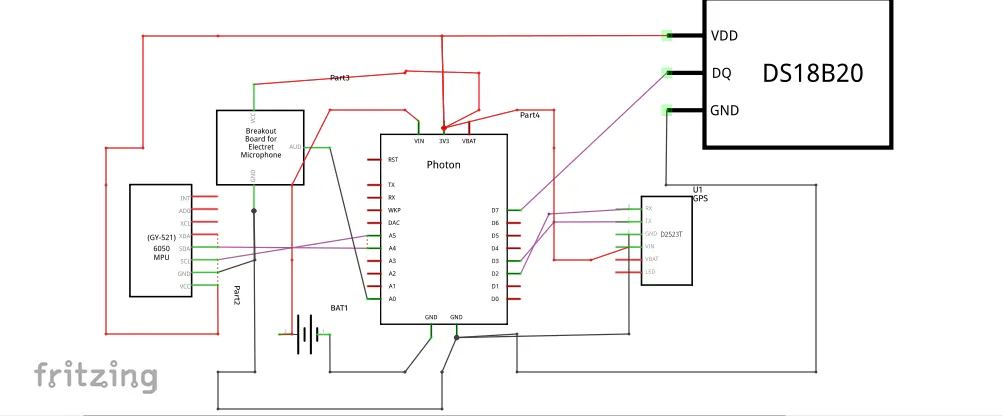## Code

### Code for the Moo Tracker

C/C++
Use this code in the particle web ide and flash it into a particle photon development board
```// This #include statement was automatically added by the Particle IDE.
#include <OneWire.h>

// This #include statement was automatically added by the Particle IDE.
#include <ParticleSoftSerial.h>

// This #include statement was automatically added by the Particle IDE.
#include <TinyGPS.h>

// This #include statement was automatically added by the Particle IDE.
#include <Ubidots.h>
// This #include statement was automatically added by the Particle IDE.
#include <OneWire.h>

#include "DS18.h"
DS18 sensor(D4);

char temp;
float current_temp = 0;
float previous_temp = 0;

/****************************************
* Define Instances and Constants
****************************************/

#ifndef UBIDOTS_TOKEN
#define UBIDOTS_TOKEN "BBFF-3yuoGIP5OsDbEBxc4q4tx33uYxlOI4"  // Put here your Ubidots TOKEN
#endif

Ubidots ubidots(UBIDOTS_TOKEN, UBI_TCP);

long accelX, accelY, accelZ;
float gForceX, gForceY, gForceZ;

long gyroX, gyroY, gyroZ;
float rotX, rotY, rotZ;

unsigned long previousMillis = 0;
const long interval = 1000;
long count = 0;
unsigned long currentMillis;
int flag = 0;
int eat;

/* This sample code demonstrates the normal use of a TinyGPS object.
It requires the use of SoftwareSerial, and assumes that you have a
4800-baud serial GPS device hooked up on pins 4(rx) and 3(tx).
*/

TinyGPS gps;
SoftwareSerial ss(2,3);

static void smartdelay(unsigned long ms);
static void print_float(float val, float invalid, int len, int prec);
static void print_int(unsigned long val, unsigned long invalid, int len);
static void print_date(TinyGPS &gps);
static void print_str(const char *str, int len);

void setup()
{
uint32_t ms = millis();
while(millis()-ms < 5000) Particle.process();
{
ms = millis();
while(millis()-ms < 60000) Particle.process();
}
Serial.begin(115200);
Particle.variable("Temerature", temp);
Wire.begin();
setupMPU();

ss.begin(9600);
}

void loop()
{
tempSensor();
recordAccelRegisters();
recordGyroRegisters();
stepCount();
delay(100);
//Particle.publish("Steps",String(count));
//delay(2000);
float flat, flon;
unsigned long age, date, time, chars = 0;
unsigned short sentences = 0, failed = 0;
static const double COIMBATORE_LAT = 11.0168, COIMBATORE_LON = 76.9558;

print_int(gps.satellites(), TinyGPS::GPS_INVALID_SATELLITES, 5);
print_int(gps.hdop(), TinyGPS::GPS_INVALID_HDOP, 5);
gps.f_get_position(&flat, &flon, &age);
print_float(flat, TinyGPS::GPS_INVALID_F_ANGLE, 10, 6);
print_float(flon, TinyGPS::GPS_INVALID_F_ANGLE, 11, 6);
print_int(age, TinyGPS::GPS_INVALID_AGE, 5);
print_date(gps);
print_float(gps.f_altitude(), TinyGPS::GPS_INVALID_F_ALTITUDE, 7, 2);
print_float(gps.f_course(), TinyGPS::GPS_INVALID_F_ANGLE, 7, 2);
print_float(gps.f_speed_kmph(), TinyGPS::GPS_INVALID_F_SPEED, 6, 2);
print_str(gps.f_course() == TinyGPS::GPS_INVALID_F_ANGLE ? "*** " : TinyGPS::cardinal(gps.f_course()), 6);
print_int(flat == TinyGPS::GPS_INVALID_F_ANGLE ? 0xFFFFFFFF : (unsigned long)TinyGPS::distance_between(flat, flon, COIMBATORE_LAT, COIMBATORE_LON) / 1000, 0xFFFFFFFF, 9);
print_float(flat == TinyGPS::GPS_INVALID_F_ANGLE ? TinyGPS::GPS_INVALID_F_ANGLE : TinyGPS::course_to(flat, flon, COIMBATORE_LAT, COIMBATORE_LON), TinyGPS::GPS_INVALID_F_ANGLE, 7, 2);
print_str(flat == TinyGPS::GPS_INVALID_F_ANGLE ? "*** " : TinyGPS::cardinal(TinyGPS::course_to(flat, flon, COIMBATORE_LAT, COIMBATORE_LON)), 6);

gps.stats(&chars, &sentences, &failed);
print_int(chars, 0xFFFFFFFF, 6);
print_int(sentences, 0xFFFFFFFF, 10);
print_int(failed, 0xFFFFFFFF, 9);
Serial.println();

smartdelay(1000);
Particle.publish("Lat",String(flat));
Particle.publish("Lon",String(flon));

/* Hardcoded Coordinates */
float latitude = flat;
float longitude = flon;

/* Reserves memory to store context key values, add as much as you need */
char* str_lat = (char*)malloc(sizeof(char) * 10);
char* str_lng = (char*)malloc(sizeof(char) * 10);

/* Saves the coordinates as char */
sprintf(str_lat, "%f", latitude);
sprintf(str_lng, "%f", longitude);

/* Reserves memory to store context array */
char* context = (char*)malloc(sizeof(char) * 30);

/* Adds context key-value pairs */

/* Builds the context with the coordinates to send to Ubidots */
ubidots.getContext(context);

/* Sends the position */
if(value1>= 1200 && value1<=1800){//(x >= 1 && x <= 100)
eat = 1;
}
else {
eat=0;
}
float value2 = count;

bool bufferSent = false;
bufferSent = ubidots.send();  // Will send data to a device label that matches the device Id

if (bufferSent) {
// Do something if values were sent properly
Serial.println("Values sent by the device");
}

/* free memory */
free(str_lat);
free(str_lng);
free(context);
//delay(5000);

// delay(5000);

}

static void smartdelay(unsigned long ms)
{
unsigned long start = millis();
do
{
while (ss.available())
} while (millis() - start < ms);
}

static void print_float(float val, float invalid, int len, int prec)
{
if (val == invalid)
{
while (len-- > 1)
Serial.print('*');
Serial.print(' ');
}
else
{
Serial.print(val, prec);
int vi = abs((int)val);
int flen = prec + (val < 0.0 ? 2 : 1); // . and -
flen += vi >= 1000 ? 4 : vi >= 100 ? 3 : vi >= 10 ? 2 : 1;
for (int i=flen; i<len; ++i)
Serial.print(' ');
}
smartdelay(0);
}

static void print_int(unsigned long val, unsigned long invalid, int len)
{
char sz;
if (val == invalid)
strcpy(sz, "*******");
else
sprintf(sz, "%ld", val);
sz[len] = 0;
for (int i=strlen(sz); i<len; ++i)
sz[i] = ' ';
if (len > 0)
sz[len-1] = ' ';
Serial.print(sz);
smartdelay(0);
}

static void print_date(TinyGPS &gps)
{
int year;
byte month, day, hour, minute, second, hundredths;
unsigned long age;
gps.crack_datetime(&year, &month, &day, &hour, &minute, &second, &hundredths, &age);
if (age == TinyGPS::GPS_INVALID_AGE)
Serial.print("********** ******** ");
else
{
char sz;
sprintf(sz, "%02d/%02d/%02d %02d:%02d:%02d ",
month, day, year, hour, minute, second);
Serial.print(sz);
}
print_int(age, TinyGPS::GPS_INVALID_AGE, 5);
smartdelay(0);
}

static void print_str(const char *str, int len)
{
int slen = strlen(str);
for (int i=0; i<len; ++i)
Serial.print(i<slen ? str[i] : ' ');
smartdelay(0);
}

//next mpu

void setupMPU(){
Wire.beginTransmission(0b1101000); //This is the I2C address of the MPU (b1101000/b1101001 for AC0 low/high datasheet sec. 9.2)
Wire.write(0x6B); //Accessing the register 6B - Power Management (Sec. 4.28)
Wire.write(0b00000000); //Setting SLEEP register to 0. (Required; see Note on p. 9)
Wire.endTransmission();
Wire.beginTransmission(0b1101000); //I2C address of the MPU
Wire.write(0x1B); //Accessing the register 1B - Gyroscope Configuration (Sec. 4.4)
Wire.write(0x00000000); //Setting the gyro to full scale +/- 250deg./s
Wire.endTransmission();
Wire.beginTransmission(0b1101000); //I2C address of the MPU
Wire.write(0x1C); //Accessing the register 1C - Acccelerometer Configuration (Sec. 4.5)
Wire.write(0b00000000); //Setting the accel to +/- 2g
Wire.endTransmission();
}

void recordAccelRegisters() {
Wire.beginTransmission(0b1101000); //I2C address of the MPU
Wire.write(0x3B); //Starting register for Accel Readings
Wire.endTransmission();
Wire.requestFrom(0b1101000,6); //Request Accel Registers (3B - 40)
while(Wire.available() < 6);
processAccelData();
}

void processAccelData(){
gForceX = accelX / 16384.0;
gForceY = accelY / 16384.0;
gForceZ = accelZ / 16384.0;
}

void recordGyroRegisters() {
Wire.beginTransmission(0b1101000); //I2C address of the MPU
Wire.write(0x43); //Starting register for Gyro Readings
Wire.endTransmission();
Wire.requestFrom(0b1101000,6); //Request Gyro Registers (43 - 48)
while(Wire.available() < 6);
processGyroData();
}

void processGyroData() {
rotX = gyroX / 131.0;
rotY = gyroY / 131.0;
rotZ = gyroZ / 131.0;
}

void stepCount()
{
if(gForceY > 0.5 && gForceY < 3.5)
{
flag =1;

previousMillis=millis();
currentMillis=millis();

}

if((currentMillis - previousMillis <= interval) && (flag))
{

if(gForceY < 0.5);
{
count++;
flag=0;

}
}
currentMillis =millis();

if(currentMillis - previousMillis > interval)
{
flag =0;

}
/*  if(count > 20)
{
count =0;
}
*/
Serial.print("steps = ");
Serial.println(count);

delay(400);

}

void tempSensor()
{
{
current_temp = sensor.celsius();
Serial.println(current_temp);
if (abs(current_temp - previous_temp) >=1)
{
snprintf(temp, sizeof(temp), "Temperature %0.2f", current_temp);
Particle.publish("Temperautre", String(sensor.celsius()),PRIVATE);
previous_temp = current_temp;
}
else
{
Serial.println("Change in Temperature is <1*C");
}
}
}
```

## Credits

### Tharrun Kumar NT

4 projects • 4 followers